﻿ 一种改进广义正交匹配追踪的DOA估计方法
«上一篇文章快速检索 高级检索

 应用科技2020, Vol. 47Issue (4): 54-58  DOI: 10.11991/yykj.2019100060

### 引用本文DIAO Hongyang, HU Zhouyong, YU Yongzhi. An improved generalized orthogonal matching pursuit method used in the direction of arrival estimation[J]. Applied Science and Technology, 2020, 47(4): 54-58. DOI: 10.11991/yykj.201910006.### 文章历史

1. 哈尔滨工程大学 信息与通信工程学院，黑龙江 哈尔滨 150001;
2. 北京遥感设备研究所，北京 100854

An improved generalized orthogonal matching pursuit method used in the direction of arrival estimation
DIAO Hongyang1, HU Zhouyong2, YU Yongzhi1
1. College of Information and Communication Engineering, Harbin Engineering University, Harbin 150001, China;
2. Beijing Institute of Remote Sensing Equipment, Beijing 100854, China
Abstract: To solve the problems of low accuracy and slow convergence rate of the DOA (direction of arrival) estimation based on traditional matching pursuit method, we propose a DOA estimation method based on improved generalized orthogonal matching pursuit (GOMP) algorithm. Firstly, a DOA estimation model is established by spatial gridding. Secondly, the GOMP algorithm is introduced to reconstruct the received signal based on this model. Specifically, the steepest descent method based on the original GOMP algorithm is introduced, and the improved GOMP algorithm is used in the DOA estimation method. Compared with the GOMP algorithm, the improved algorithm employs the steepest descent method with simple operation to perform signal reconstruction, reduces complexity of the reconstruction algorithm. The simulation results demonstrate that the improved algorithm has successfully obtained the DOA estimation value. Compared with the traditional OMP algorithm, the improved algorithm can obtain higher DOA estimation accuracy, which effectively reduces the reconstruction time and has higher estimation performance.
Keywords: DOA estimation    array signal processing    compressed sensing    greedy algorithm    sparse representation    generalized orthogonal matching pursuit    steepest descent method    least square method

1 基于稀疏表示的DOA估计模型

 ${{y}}(t) = {{Ax}}(t) + {{n}}(t)$ (1)
 ${{A}} = \left[ {{{a}}\left( {{\theta _1}} \right),{{a}}\left( {{\theta _2}} \right), \cdots ,{{a}}\left( {{\theta _N}} \right)} \right]$ (2)
 ${{a}}\left( {{\theta _n}} \right) = {\left[ {1,{{\rm{e}}^{{\rm{j}}{\varphi _1}}}, \cdots ,{{\rm{e}}^{{\rm{j}}(M - 1){\varphi _n}}}} \right]^{\rm{T}}}$
 ${\varphi _n} = 2{\text{π}}d\sin {\theta _n}/\lambda ,n \in \left\{ {1,2, \cdots ,N} \right\}$

2 基于改进GOMP算法的DOA估计 2.1 OMP算法

2.2 GOMP算法

OMP算法和GOMP算法均是通过贪婪迭代从阵列流型矩阵 ${{A}}$ 中找到能够提供最大能量的某些列原子，代价函数选用 ${{y}}$ 残差最小。2种算法的区别在于进行重构时，OMP算法每次迭代过程中只选择与 ${{y}}$ 中剩余部分最相关的一列，而GOMP算法在每次迭代过程中会选择与 ${{y}}$ 中剩余部分最相关的 $K$ 列，然后在所有选出的可能的坐标集合中进行搜寻，在索引坐标集合中加入使估计能量值最大的坐标。相对于OMP算法，GOMP算法的搜索选择方式能够更好地保证从信号能量相近或者不完全和不精确的阵列接收信号中恢复信号，能更准确地找出信号源所在的真实角度位置。

2.3 最速下降法的相关理论

 $f({{x}}) = f({{{x}}_k}) + \nabla f{({{{x}}_k})^{\rm{T}}}({{x}} - {{{x}}_k}) + o({{x}})$

 $\left| {{{d}}_k^{\rm{T}}{{{g}}_k}} \right| \leqslant \left| {{{{d}}_k}} \right|\left| {{{{g}}_k}} \right|$

 ${a_k} = \arg \min f\left( {{{{x}}_k} - a{{{g}}_k}} \right)$

 ${{{x}}_{k + 1}} = {{{x}}_k} - a{{{g}}_k}$

 $f({{x}}) = \frac{1}{2}{{{x}}^{\rm{T}}}{{Q}}{{x}} - {{{b}}^{\rm{T}}}{{x}}$

 ${{{g}}_k} = \nabla f({{x}}) = {{Q}}{{x}} - {{b}}$

 ${{{x}}_{k + 1}} = {{{x}}_k} - a{{{g}}_k}$

 ${a_k} = \arg \min f\left( {{{{x}}_k} - a{{{g}}_k}} \right)$

${{{g}}_k} = 0$ 时迭代过程停止，在不为零时，设 ${\varphi _k}(a) = f({{{x}}_k} - a{{{g}}_k})$ ，由局部最小点的一阶必要条件得到：

 ${{{\varphi}}'}_k(a) = {({{{x}}_k} - a{{{g}}_k})^{\rm{T}}}{{Q}}( - {{{g}}_k}) - {{{b}}^{\rm{T}}}( - {{{g}}_k})$

${{{\varphi}}'}_k(a) = 0$ 时， $a{{g}}_k^{\rm{T}}{{Q}}{{{g}}_k}{\rm{ = (}}{{x}}_k^{\rm{T}}{{Q}} - {{{b}}^{\rm{T}}}){{{g}}_k}$ ，因为 ${{Q}}$ 是对称正定阵， ${{Q}} = {{{Q}}^{\rm{T}}}$ ${{x}}_k^{\rm{T}}{{Q}} - {{{b}}^{\rm{T}}} = {{g}}_k^{\rm{T}}$ ，由此可以得到步长 ${a_k} = \dfrac{{{{g}}_k^{\rm{T}}{{{g}}_k}}}{{{{g}}_k^{\rm{T}}{{Q}}{{{g}}_k}}}$ ${a_k}$ 即为最速下降的步长。

2.4 改进GOMP算法

${{\varPhi}} = \left\{ {{\varphi _k}} \right\}$ 表示测量矩阵，此测量矩阵即为DOA估计模型中的阵列流型矩阵， ${{y}}$ 表示要恢复的信号， ${{{x}}_i}$ 表示第 $i$ 次循环得到的近似信号， ${{{r}}_i}$ 表示残差， ${{{\varLambda}} _i}$ 表示索引集合， ${{\varPsi}}$ 表示选择原子的列向量集合，先得到矩阵的负梯度方向为 ${{{g}}_i} = {{{\varPsi}} _i}^{\rm{T}}{{{r}}_i}$ ，然后计算每次迭代的步长为

 $\begin{array}{c} {a_i} = \dfrac{{\left\langle {{{{g}}_i},{{{g}}_i}} \right\rangle }}{{\left\langle {{{{g}}_i},{{{\varPsi }}_i}^{\rm{T}}{{{\varPsi }}_i}{{{g}}_i}} \right\rangle }} = \dfrac{{\left\langle {{{{\varPsi }}_i}^{\rm{T}}{{{r}}_i},{{{g}}_i}} \right\rangle }}{{\left\langle {{{{g}}_i},{{{\varPsi }}_i}^{\rm{T}}{{{\varPsi }}_i}{{{g}}_i}} \right\rangle }} =\\ \dfrac{{\left\langle {{{{r}}_i},{{{c}}_i}} \right\rangle }}{{\left\langle {{{{\varPsi }}_i}{{{g}}_i},{{{\varPsi }}_i}{{{g}}_i}} \right\rangle }} = \dfrac{{\left\langle {{{{r}}_i},{{{c}}_i}} \right\rangle }}{{\left\| {{{{c}}_i}} \right\|_2^2}} \end{array}$ (3)

 ${{{x}}_i} = {{{x}}_{i - 1}} + {a_i}{{{g}}_i}$

 ${{{r}}_i} = {{{r}}_{i - 1}} - {a_i}{{{c}}_i}$ (4)

1)初始化残差 ${{{r}}_0} = {{y}}$ ，初始化索引集 $\varLambda$ 为空集，初始化迭代次数 $i = 1$

2)计算残差和测量矩阵 ${{\varPhi}}$ 的每一列的内积，找出若干个绝对值最大的元素，并将这些值对应的列序号构成集合 ${{P}}$

3)更新索引集 ${\varLambda _i} = {\varLambda _{i - 1}} \cup P$ ，更新列向量集合 ${{{\varPsi}} _i} = {{{\varPsi}} _{i - 1}} \cup {{{\varphi }} _P}$

4)利用 ${{{g}}_i} = {{{\varPsi}} _i}^{\rm{T}}{{{r}}_i}$ 计算负梯度方向 ${{{g}}_i}$ ，利用式(3)计算步长 ${a_i}$

5)利用式(4)更新残差；

6)若满足迭代停止条件，根据索引集 $\varLambda$ 在角度 ${\theta _{\rm{i}}}(i = 1,2, \cdots ,N)$ 中找到信号源所在的真实角度位置；若不满足，则跳转到步骤2)进行下一次循环。

3 仿真结果分析

 ${\rm{RMSE}} = \sqrt {\frac{1}{{MK}}{{\sum\limits_{m = 1}^M {\sum\limits_{k = 1}^K {\left( {{{\hat \theta }_{km}} - {\theta _{km}}} \right)^2} } }}}$ (5)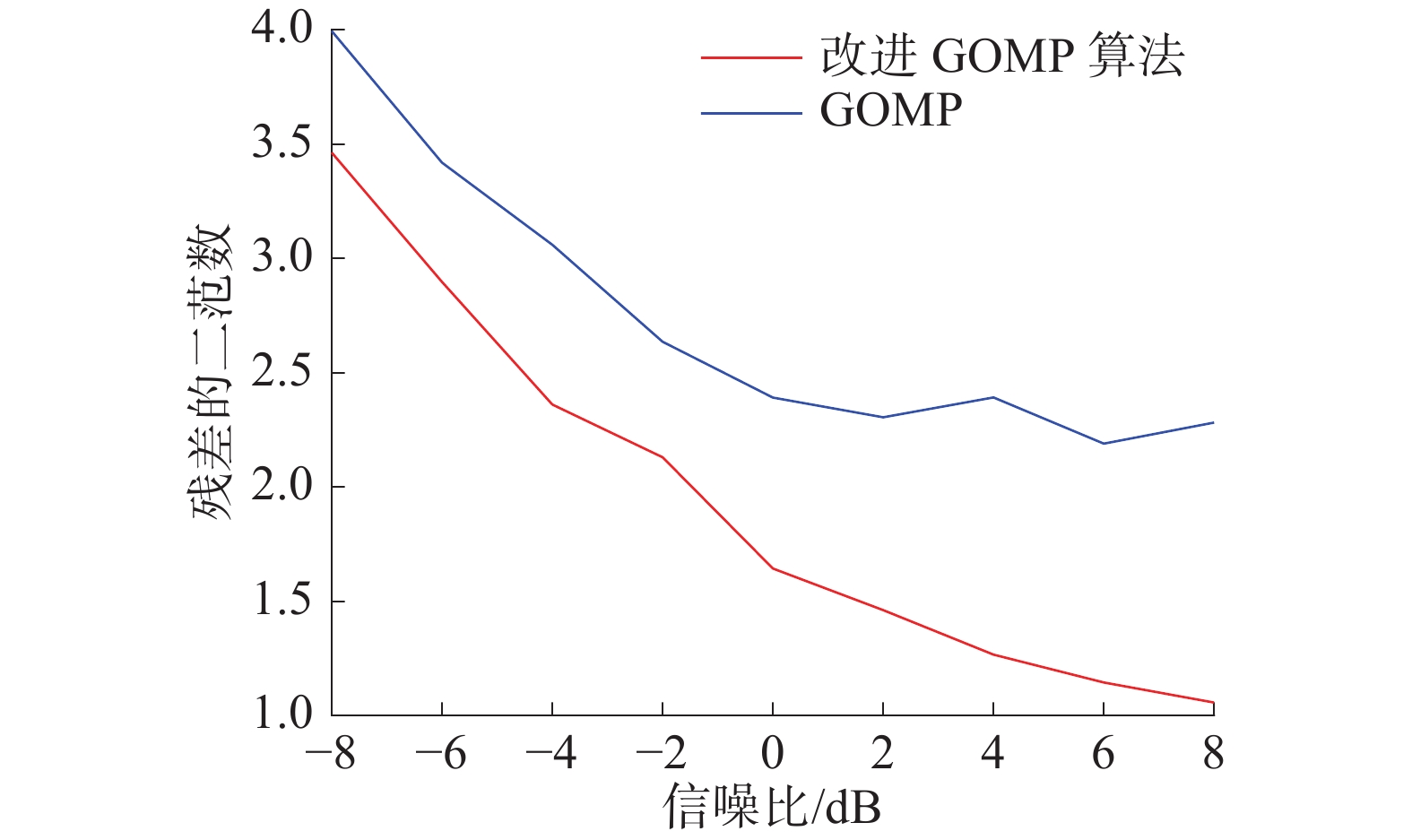Download: 图 1 改进GOMP算法与GOMP算法残差比较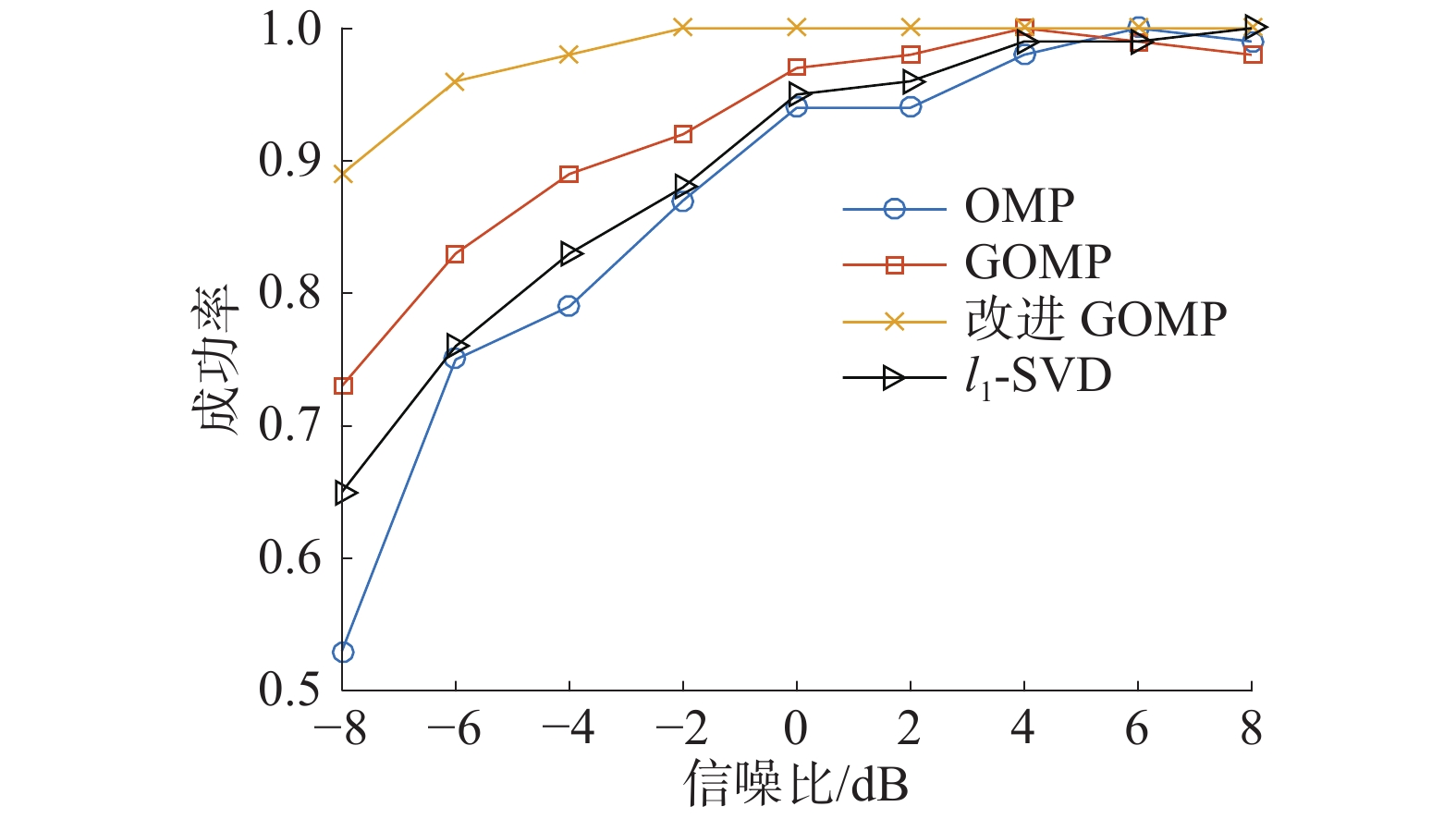Download: 图 2 不同信噪比下DOA估计成功概率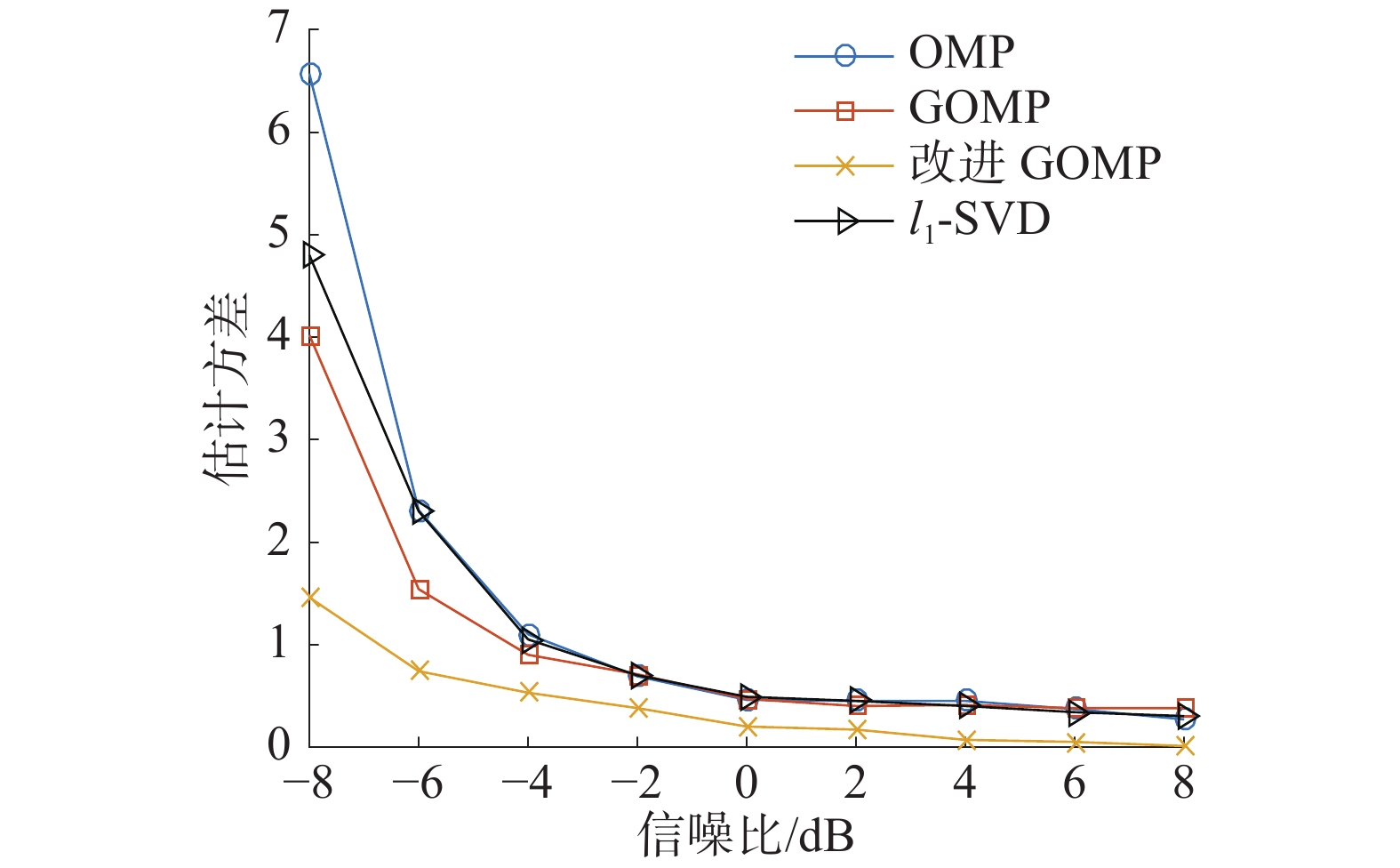Download: 图 3 不同信噪比下DOA估计均方根误差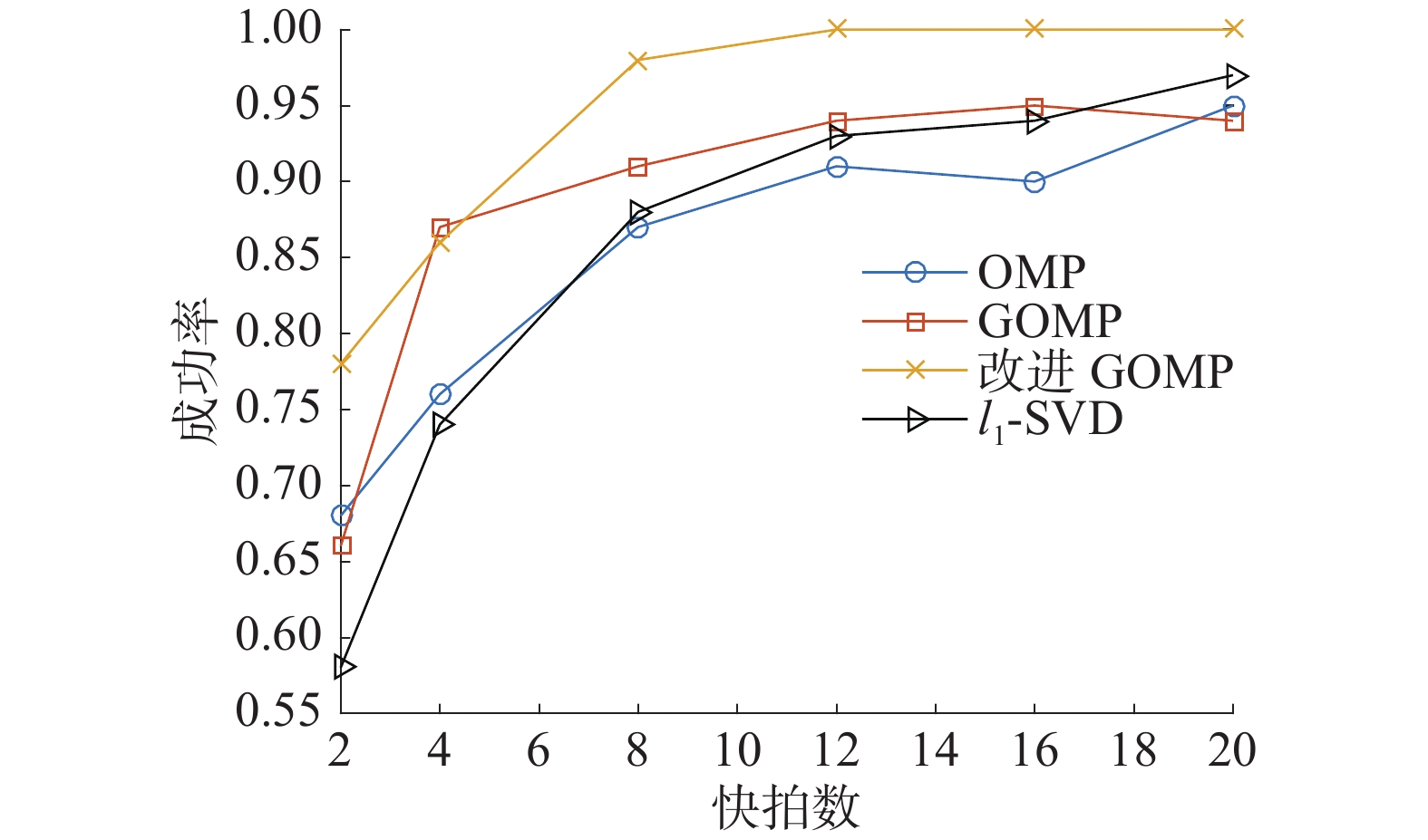Download: 图 4 不同快拍数下DOA估计成功率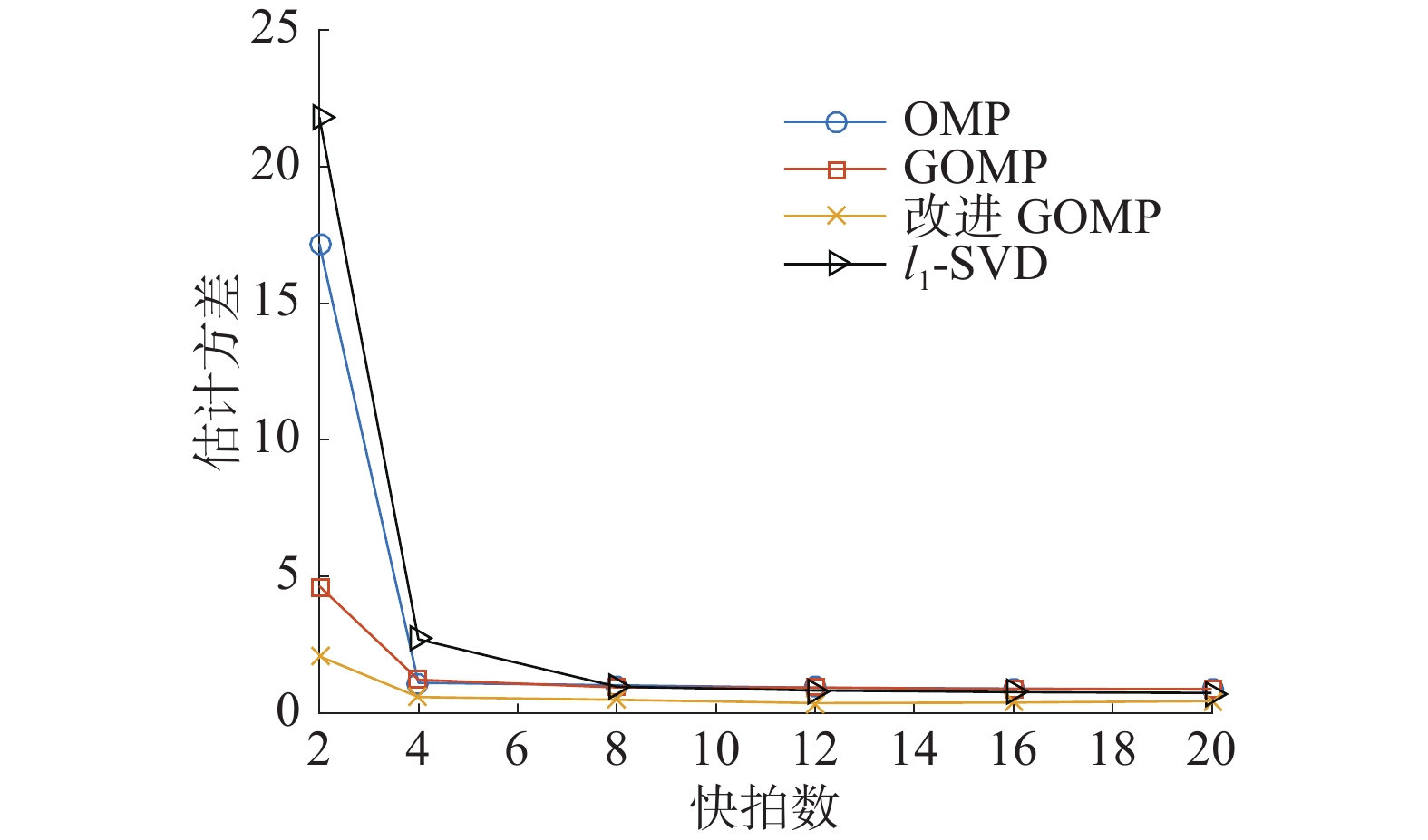Download: 图 5 不同快拍数下DOA估计均方根误差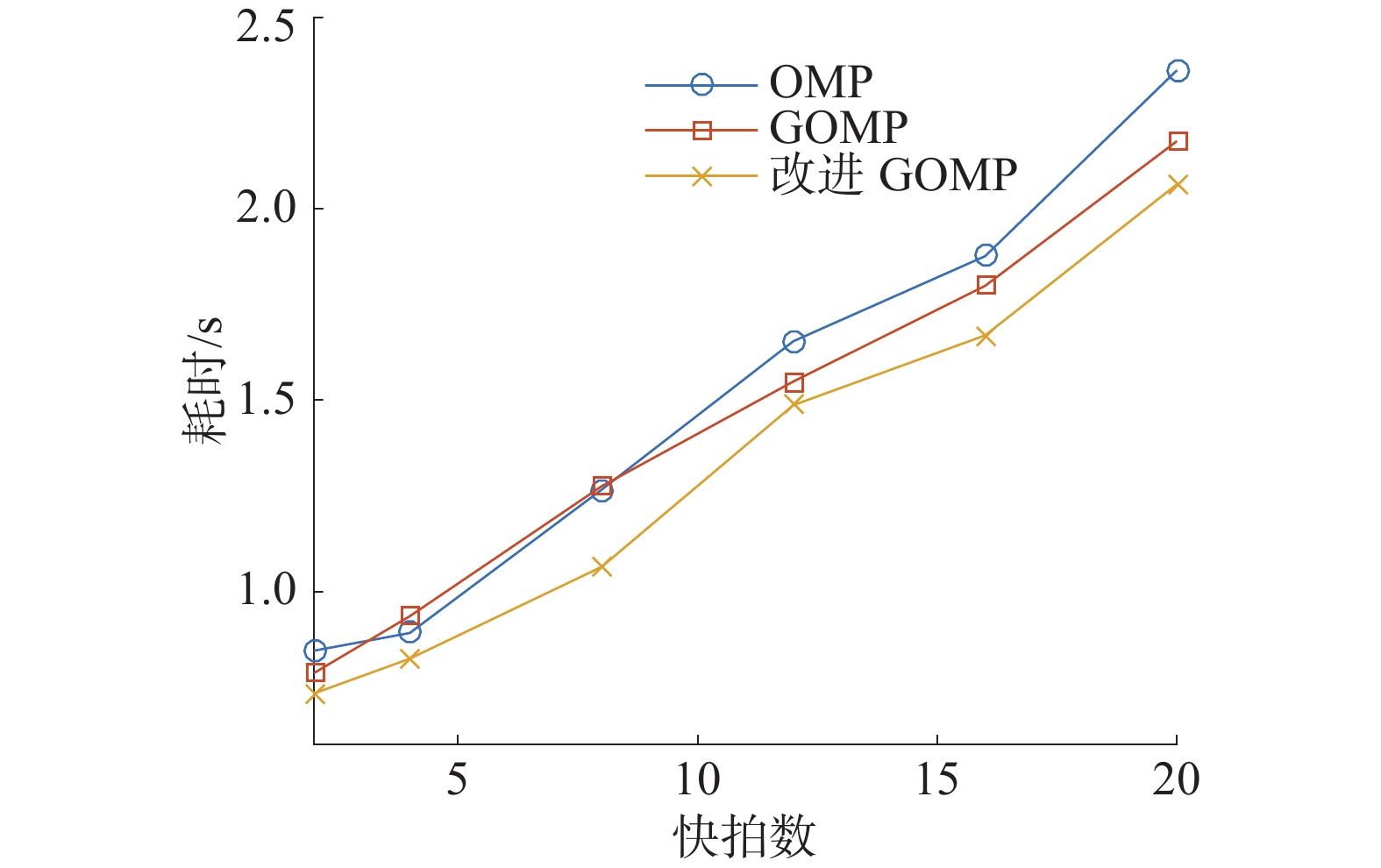Download: 图 6 不同快拍数下算法运行时间比较
4 结论

1)利用GOMP算法求解DOA问题，相较于原有贪婪DOA估计方法提高了估计精度和成功率。

2)引入最速下降法对GOMP算法进行优化，并通过仿真验证了改进方法具有更高的估计精度和成功率，降低了算法的运行时间，相对于原有贪婪DOA估计方法的性能有一定的提升。

  汤永涛, 林鸿生, 陈春. 现代导弹导引头发展综述[J]. 制导与引信, 2014(1): 12-17. DOI:10.3969/j.issn.1671-0576.2014.01.003 (0)  王永良, 陈辉, 彭应宁. 空间谱估计理论与算法[M]. 北京: 清华大学出版社, 2004. (0)  张小飞. 阵列信号处理的理论与应用[M]. 北京: 国防工业出版社, 2013. (0)  SCHMIUDT R. Multiple emitter location and signal parameter estimation[J]. IEEE transactions on antennas and propagation, 1986, 34(3): 276-280. DOI:10.1109/TAP.1986.1143830 (0)  ROY R, KAILATH T. ESPRIT-estimation of signal parameters via rotational invariance techniques[J]. IEEE transactions on acoustics speech and signal processing, 1989, 37(7): 984-995. DOI:10.1109/29.32276 (0)  JIANG Guojun, MAO Xingpeng, LIU Yongtan. Reducing errors for root-MUSIC-based methods in uniform circular arrays[J]. IET signal processing, 2018, 12(1): 31-36. DOI:10.1049/iet-spr.2016.0576 (0)  LIU Lutao, LIU Huan. Joint estimation of DOA and TDOA of multiple renections in mobile communications[J]. IEEE access, 2016, 4: 3815-3823. (0)  YAN Fenggang, LIU Shuai, WANG Jun. Real-valued root-MUSIC for DOA estimation with reduced-dimension EVD/SVD computation[J]. Signal processing, 2018, 152: 1-12. DOI:10.1016/j.sigpro.2018.05.009 (0)  DONOBO D L. Compressed sensing[J]. IEEE transactions on information theory, 2006, 52(4): 1289-1306. DOI:10.1109/TIT.2006.871582 (0)  WEI Jingbo, HUANG Yukun, KE Lu. Nonlocal low-rank-based compressed sensing for remote sensing image reconstruction[J]. IEEE geoscience and remote sensing letters, 2017, 13(10): 1557-1561. (0)  冯俊杰, 张弓, 文方青. 基于SL0范数的改进稀疏信号重构算法[J]. 数据采集与处理, 2016, 31(1): 178-183. (0)  单泽涛, 刘小松, 单泽彪, 等. 基于改进平滑l0范数的DOA估计算法 [J]. 吉林大学学报(信息科学版), 2017(6): 22-26. (0)  MALIOUTOV D, CETIN M, WILLSKY A S. A sparse signal reconstruction perspective for source localization with sensor arrays[J]. IEEE transactions on signal processing, 2005, 53(8): 3010-3022. DOI:10.1109/TSP.2005.850882 (0)  燕学智, 温艳鑫, 刘国红. 基于稀疏表示和近似l0范数约束的宽带信号DOA估计 [J]. 航空学报, 2017(6): 221-228. (0)  刘晓, 吴明儿, 张华振. 基于最速下降法的可展开索网天线型面调整方法[J]. 中国空间科学技术, 2018, 38(3): 1-7. (0)  TROPP J A, GILBERT A C. Signal recovery from random measurements via orthogonal matching pursuit[J]. IEEE transactions on information theory, 2007, 53(12): 4655-4666. DOI:10.1109/TIT.2007.909108 (0)  NEEDELL D, VERSKYNIN R. Signal recovery from incomplete and inaccurate measurements via regularized orthogonal matching pursuit[J]. IEEE journal of selected topics in signal processing, 2010, 4(2): 310-316. DOI:10.1109/JSTSP.2010.2042412 (0)  蔡盛盛, 张佳维, 冯大航. 改进正则化正交匹配追踪波达方向估计方法[J]. 声学学报, 2014(1): 39-45. (0)  王军, 孔令斌, 赵洁. 基于压缩感知的OMP改进重构算法[J]. 光通信研究, 2016(1): 74-78. (0)  DEHGHANI M, AGHABABAIYAN K. FOMP algorithm for direction of arrival estimation[J]. Physical communication, 2018, 26: 170-174. DOI:10.1016/j.phycom.2017.12.012 (0)